# 14-240/Classnotes for Monday September 8

We went over "What is this class about?" (PDF, HTML), then over "About This Class", and then over the first few properties of real numbers that we will care about.

 Dror's notes above / Students' notes below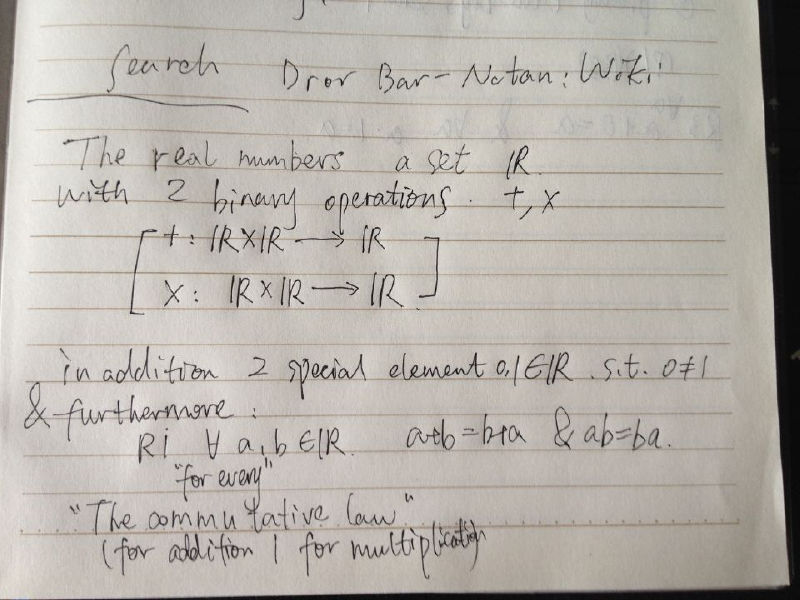===The Real Numbers===

The real numbers are a set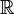$\R$ with 2 binary operations + and *, defined as follows: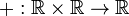$+: \R \times \R \rightarrow \R$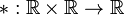$*: \R \times \R \rightarrow \R$


in addition to 2 special elements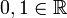$0, 1 \in \R$ such that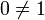$0 \ne 1$, with the following properties: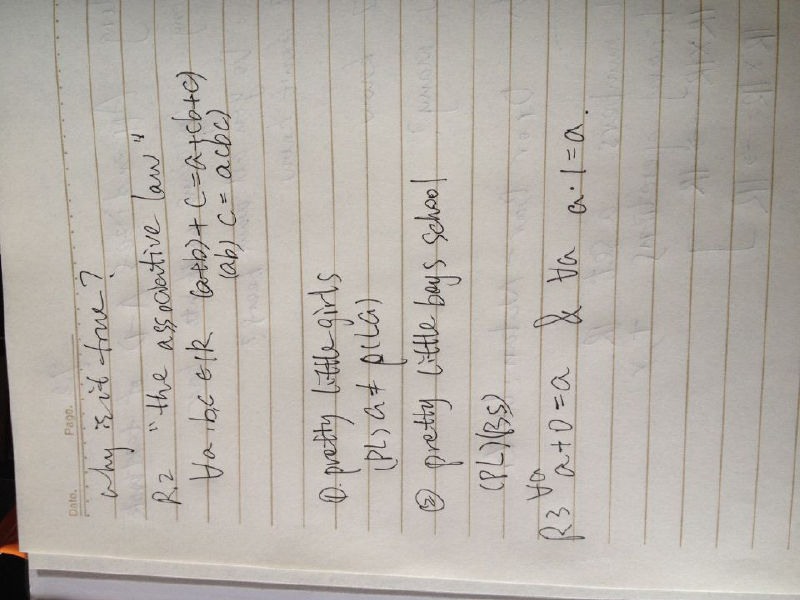====The Commutative Law====

R1: For every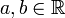$a, b \in \R$, we have: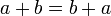$a + b = b + a$ (commutative law for addition)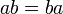$ab = ba$ (commutative law for multiplication)


#### The Associative Law

R2: For every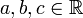$a, b, c \in \R$, we have: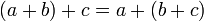$(a + b) + c = a + (b + c)$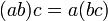$(ab)c = a(bc)$


This is not true for a number of other sets in our lives! For example, the associative law does not hold for the English language. Consider the phrase "pretty little girls": "(pretty little) girls" does not mean the same thing as "pretty (little girls)".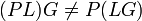$(PL)G \ne P(LG)$


So the associative property does not hold for the English language.

#### Existence of Units

R3: For every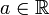$a \in \R$: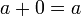$a + 0 = a$ (additive unit)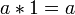$a * 1 = a$ (multiplicative unit)


## Wednesday September 10th 2014 - Fields

The real numbers: A set |R with +,x : |R x |R -> |R &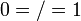$0=/=1$ are elements of |R such that

R1: For every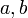$a, b$ that are elements of |R ,$a + b = b + a$ and$ab = ba$

R2: For every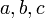$a, b, c$ that are elements of |R,$( a + b ) + c = a + ( b + c )$ and$(ab)c = a(bc)$

R3: For every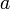$a$ that is an element of |R,$a + 0 = a$ and$a * 1 = a$

R4: For every a that is an element of |R there exists b that is an element of |R such that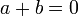$a + b = 0$ & for every a that is an element of |R and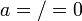$a =/= 0$ there exists b that is an element |R such that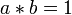$a * b = 1$

R5: For every$a, b, c$ that are elements of |R,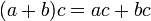$( a + b ) c = ac + bc$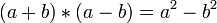$( a + b ) * ( a - b ) = a^2 - b^2$ follows from R1-R5

The following is true for the Real Numbers but does not follow from R1-R5 For every a that is an element of |R there exists an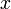$x$ that is an element of |R such that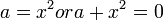$a = x^2 or a + x^2 = 0$

However we can see that it does not follow from R1-R5 because we can find a field that obeys R1-R5 yet does not follow the above rule. An example of this is the Rational Numbers |Q. In |Q take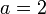$a = 2$ and there does not exist$x$ such that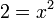$2 = x^2$ or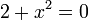$2 + x^2 = 0$

The Definition Of A Field: A "Field" is a set F along with a pair of binary operations +,x : FxF -> F and along with a pair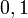$0, 1$ that are elements of F such that$0 =/= 1$ and such that R1-R5 hold.

R1: For every$a, b$ that are elements of F ,$a + b = b + a$ and$ab = ba$

R2: For every$a, b, c$ that are elements of F,$( a + b ) + c = a + ( b + c )$ and$(ab)c = a(bc)$

R3: For every$a$ that is an element of F,$a + 0 = a$ and$a * 1 = a$

R4: For every$a$ that is an element of F there exists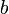$b$ that is an element of F such that$a + b = 0$ & for every$a$ that is an element of F and$a =/= 0$ there exists$b$ that is an element F such that$a * b = 1$

R5: For every$a, b, c$ that are elements of F,$( a + b ) c = ac + bc$

Example

1. |R is a field (real numbers) 2. |Q is a field (rational numbers) 3. |C is a field (complex numbers) 4. F = {0, 1}

• insert table of addition and multiplication*

Proposition: F is a Field checking F5

etc...

F = {0 , 1} = F2 = Z/2

Do the same for F7

• insert table of addition and multiplication*

"Like remainders when you divide by 7" "like remainders mod 7'

Theorem (that shall remain unproved) : For every prime number P, FP = {0 , 1 , 2 , ... , p-1 } along with + & x defined as above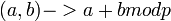$( a , b ) -> a + b mod p$ is a field.

Theorem: (basic properties of Fields)

Let F be a Field, and let a , b , c denote elements of F Then: 1.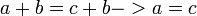$a + b = c + b -> a = c$ "Cancellation" still holds 2.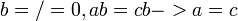$b =/= 0 , ab = cb -> a = c$ 3. If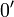$0'$ is an element of F and satisfies for every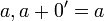$a , a + 0' = a$ , then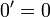$0' = 0$ 4. If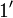$1'$ is "like 1" then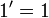$1' = 1$

... to be continued...